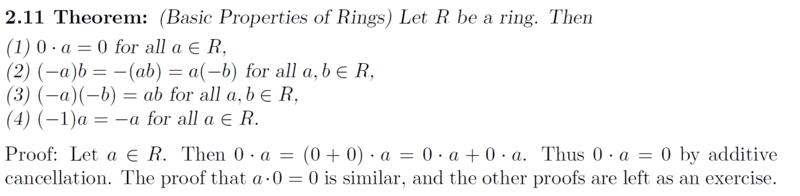# Prove the given properties - Ring Theory

• I
• chwala

#### chwala

Gold Member
TL;DR Summary
I am looking at the basics here...Consider the attachment below. My intention is to try and prove ##2.11## from ##2## to ##4## and i would appreciate any constructive feedback.Ok for ##1##, we also have,
##a⋅0=a⋅(0+0)=a⋅0 + a⋅0 ## We know that ##a⋅0=0 ## by additive cancellation.

For ##2.11##, Number ##2##;
We first show and prove that
##-b=-1⋅b##
##-b+b=0## for the lhs
##-1⋅b +1⋅b=b(-1+1)=b(0)=0## for the rhs
therefore,
##(-a)b=(-1⋅a)b=-1(ab)=-(ab)## using distributive property...
also,
##a⋅(-b)=a⋅-1⋅b=-1⋅(a⋅b)=-1(ab)=-(ab)##
Will look at (3) and (4) later...

For number 2) you need to show that, for example:
$$(-a) b + a b = a b + (-a) b = 0$$Hence, by definition:$$(-a)b = -(ab)$$ is the additive inverse of ##ab##.

•chwala
So in general, we are just checking as to whether the ring additive axioms are satisfied, right?...I can see that you have used commutative property and the additive inverse property to show this...in my case I could say that I made use of the distributive property...
In showing these proofs, there isn't any general rule right? The way is to check how the problem fits/satisfies the ring property axioms...

I also read on the Field which basically has more axioms satisfied ( ie For Real, rational and complex numbers)as compared to the Ring. (Integers)...

The problem with what you did was that you didn't fully recognise that ##-(ab)## is, by notational definition, the additive inverse of ##ab##. And that we have to show that ##(-a)b##, which is the additive inverse of ##a## multiplied by ##b##, is also the additive inverse of ##ab##.

The problem with what you did was that you didn't fully recognise that ##-(ab)## is, by notational definition, the additive inverse of ##ab##. And that we have to show that ##(-a)b##, which is the additive inverse of ##a## multiplied by ##b##, is also the additive inverse of ##ab##.
Thanks, yes that's true...I can see that now.

@PeroK hi, by the way could i also be right in using,
if ##a=b## and ##b=c## then it follows that, ##a=c##
##a-b=0## and ##b-c=0##, ##⇒a-b+b-c=0##

therefore in our problem we shall have,
##(-a)b=-(ab)##
##(-a)b- - (ab)=0##

also,
##-(ab)=a(-b)##
##-(ab)-a(-b)=0##,

##(-a)b- -(ab)+-(ab)-a(-b)=0##
##(-a)b+(ab)-(ab)-a(-b)=0##
##(-a)b-a(-b)=0##
##(-a)b=a(-b)## thus completing our proof.

therefore in our problem we shall have,
##(-a)b=-(ab)##
##(-a)b- - (ab)=0##
You can't start with what you are asked to prove. Try starting with $$(-a)b + ab = \dots$$

You can't start with what you are asked to prove. Try starting with $$(-a)b + ab = \dots$$
ok, i may have, ##(-a)b+ab=b(-a+a)=b⋅0=0##
also, ##-(ab)+ab=0##
also,##(a)-b+ab=a(-b+b)=a⋅0=0## therefore ##(-a)b=-(ab)=(a)-b##

Last edited:
ok, i may have, ##(-a)b+ab=b(-a+a)=b⋅0=0##
A ring is not necessarily commutative, so you can't swap the order like that:$$(-a)b+ab=(-a+a)b=(0)b=0$$
also, ##-(ab)+ab=0## ##⇒(-a)b=-(ab)##
That's not quite right either. $$(-a)b+ab = 0 \ \Rightarrow \ (-a)b = -(ab)$$
also,##(a)-b+ab=a(-b+b)=a⋅0=0## therefore our proof is complete.
Okay, but ##a(-b)## is better than ##(a)-b##

•chwala
A ring is not necessarily commutative, so you can't swap the order like that:$$(-a)b+ab=(-a+a)b=(0)b=0$$

That's not quite right either. $$(-a)b+ab = 0 \ \Rightarrow \ (-a)b = -(ab)$$

Okay, but ##a(-b)## is better than ##(a)-b##
cheers gd day...

cheers gd day...
It is abstract algebra; not slapdash algebra!•chwala
A ring is not necessarily commutative, so you can't swap the order like that:$$(-a)b+ab=(-a+a)b=(0)b=0$$

That's not quite right either. $$(-a)b+ab = 0 \ \Rightarrow \ (-a)b = -(ab)$$

Okay, but ##a(-b)## is better than ##(a)-b##
noted on this; $$(-a)b+ab=(-a+a)b=(0)b=0$$ thanks...i will read more on that...i had thought that for a set to be considered a ring, then it has to satisfy commutativity...which you are saying it is not always the case...

noted on this; $$(-a)b+ab=(-a+a)b=(0)b=0$$ thanks...i will read more on that...i had thought that for a set to be considered a ring, then it has to satisfy commutativity...which you are saying it is not always the case...
Ring multiplication is not necessarily commutative. Ring addition is commutative, of course.

•chwala
For ##3##,
We want to prove,
##(-a)(-b) =ab \\∀ a,b ∈ \mathbb{R}##

Using distributive property and using ##-(ab)## on lhs;
##(-a)(-b) -(ab)=-(a)(-b)-(ab)=[--(ab)]-(ab)=(ab)-(ab)=0##

Also using ##-(ab)## on rhs;
##ab-(ab)=ab - ab=0##,

therefore,
##(-a)(-b) =ab ∀ a, b ∈ \mathbb{R}##

Last edited:
For ##4##,
We want to prove,
##(-1)(a) =-a \\∀ a ∈ \mathbb{R}##

For lhs, using distributive property;
##(-1)a + 1a=(-1+1)a=(0)a=0##
also for rhs, ##-a=-(1a)##
##⇒(-1)a- -a=(-1)a+a=(-1)a+1a=0##
thus ##(-1)a=-a##